﻿ 一种多样性和精度加权的数据流集成分类算法
«上一篇文章快速检索 高级检索

 智能系统学报2019, Vol. 14Issue (1): 179-185  DOI: 10.11992/tis.2018060210

### 引用本文ZHANG Bencai, WANG Zhihai, SUN Yan’ge. An ensemble classification algorithm based on diversity and accuracy weighting for data streams[J]. CAAI Transactions on Intelligent Systems, 2019, 14(1): 179-185. DOI: 10.11992/tis.201806021.### 文章历史

1. 北京交通大学 计算机与信息技术学院，北京 100044;
2. 信阳师范学院 计算机与信息技术学院，河南 信阳 464000

An ensemble classification algorithm based on diversity and accuracy weighting for data streams
ZHANG Bencai 1, WANG Zhihai 1, SUN Yan’ge 1,2
1. School of Computer and Information Technology, Beijing Jiaotong University, Beijing 100044, China;
2. School of Computer and Information Technology, Xinyang Normal University, Xinyang 464000, China
Abstract: To overcome the effect of concept drift on data stream classification, we propose an ensemble classification algorithm based on diversity and accuracy weighting named DAWE. The difference between DAWE and other existing ensemble methods is that DAWE considers both diversity and accuracy. The classifier’s accuracy on the new data chunk and its diversity in the ensemble were linearly weighted to measure the value of the current ensemble classifier and the measured value was applied to the substitute strategy of the base classifier. The DAWE algorithm proposed in this paper was experimentally compared with the latest algorithms in massive online analysis (MOA), using both synthetic and real-world datasets. Experiments showed that the method proposed in this paper was effective and the average overall accuracy of the data sets was superior to that of other algorithms. Overall, this method can effectively manage concept drift in data stream mining.
Key words: data stream    concept drift    diversity    accuracy    ensemble learning    data chunk    value measurement    MOA

1 相关工作

2 加权多样性和精度的集成方法

2.1 分类器的精度和多样性度量

1) 精度度量

 ${\rm{MS}}{{\rm{E}}_{ij}} = \frac{1}{{\left| {{B_j}} \right|}}{\sum\limits_{\{ {{x}},y\} \in {B_j}} {(1 - p({f_i}({{x}}) = y))} ^2}$ (1)

 ${\rm{MS}}{{\rm{E}}_r} = {\sum\limits_y {p(y)(1 - p(y))} ^2}$ (2)

 ${\rm{Ac}}{{\rm{c}}_{ij}} = \frac{1}{{{\rm{MS}}{{\rm{E}}_{ij}} + \delta }}$ (3)

 ${\rm{Ac}}{{\rm{c}}_{C'}} = \frac{1}{{{\rm{MSE}}{}_r + \delta }}$ (4)

2) 集成分类器中的多样性度量

 ${Q_{ij}} = \frac{{{N^{11}}{N^{00}} - {N^{01}}{N^{10}}}}{{{N^{11}}{N^{00}} + {N^{01}}{N^{10}}}}$ (5)

 $Q_{ij}^* = 0.5(1 - {Q_{ij}})$ (6)

 ${\rm{di}}{{\rm{v}}_{{{C}}'}} = \sum\limits_{i = C'\!\!,\;{C_j} \in E} {{{Q}}_{ij}^*} /\left| E \right|$ (7)

 ${\rm{di}}{{\rm{v}}_i} = \sum\limits_{{C_j} \in E,i \ne j} {{{Q}}_{ij}^*} /\left| E \right|$ (8)
2.2 基于多样性和精度加权的集成算法

1)基于多样性和精度加权的分类器权重计算

 ${w_{{{C}}'}} = \alpha {\rm{Ac}}{{\rm{c}}_{C'}} + (1 - \alpha ){\rm{di}}{{\rm{v}}_{C'}}$ (9)

 ${w_{ij}} = \alpha {\rm{Ac}}{{\rm{c}}_{ij}} + (1 - \alpha ){\rm{di}}{{\rm{v}}_i}$ (10)

2)基于价值度量的集成分类器更新策略

3) 算法过程

1) begin

2)将E初始为空；

3)对于数据流S的每个到来的数据块Bi

4)在数据块Bi上训练新分类器C′；

5)由式(9)计算C′的权重；

6)对于每个E中的分类器Cj

7)计算 Cj的精度(由式(3))；

8)由式(8)计算 Cj的多样性；

9)由式(10)计算Cj的权重；

10)如果E中分类器个数小于n；将C′直接添加到E中；

11)否则使用C′替换E中权重最低的分类器；

12)对于E中除去C′之外的基分类器Cj

13)在数据块Bi上增量训练 Cj

14) end

3 实验

3.1 数据集

3.1.1 真实数据集

1)扑克牌(Poker)数据集：来源于UCI数据库，每个实例有11个属性。数据集中每个实例由52张牌中的5张组成，每张牌使用两个属性(suit和rank)来描述。

2)Covertype数据集：来自UCI数据库，该数据集包含了4个野生区域覆盖类型信息。该数据集有581 012个实例，每个实例有53个属性对应7种可能的森林覆盖类型中的1种。

3)Airlines数据集：该数据集包含根据航班的出发信息来预测此次航班是否会晚点的数据。此数据集包含539 383个实例，每个实例包含7个属性。

3.1.2 合成数据集

1)SEA数据集：该数据集是Street于2001年提出的，因仅含有连续型属性而著名，是经典的突变式概念漂移数据集。

2)LED数据集：该数据集用来预测7段数码显示器上显示的数字。该数据集有24个属性，其中前7个属性用于显示0~9的数字。

3)随机树数据集：该数据集由5个nominal属性和5个numeric属性组成，类属性值通过随机树(random tree)确定。

3.2 实验结果对比与分析

3.2.1 不同数据块大小对算法性能影响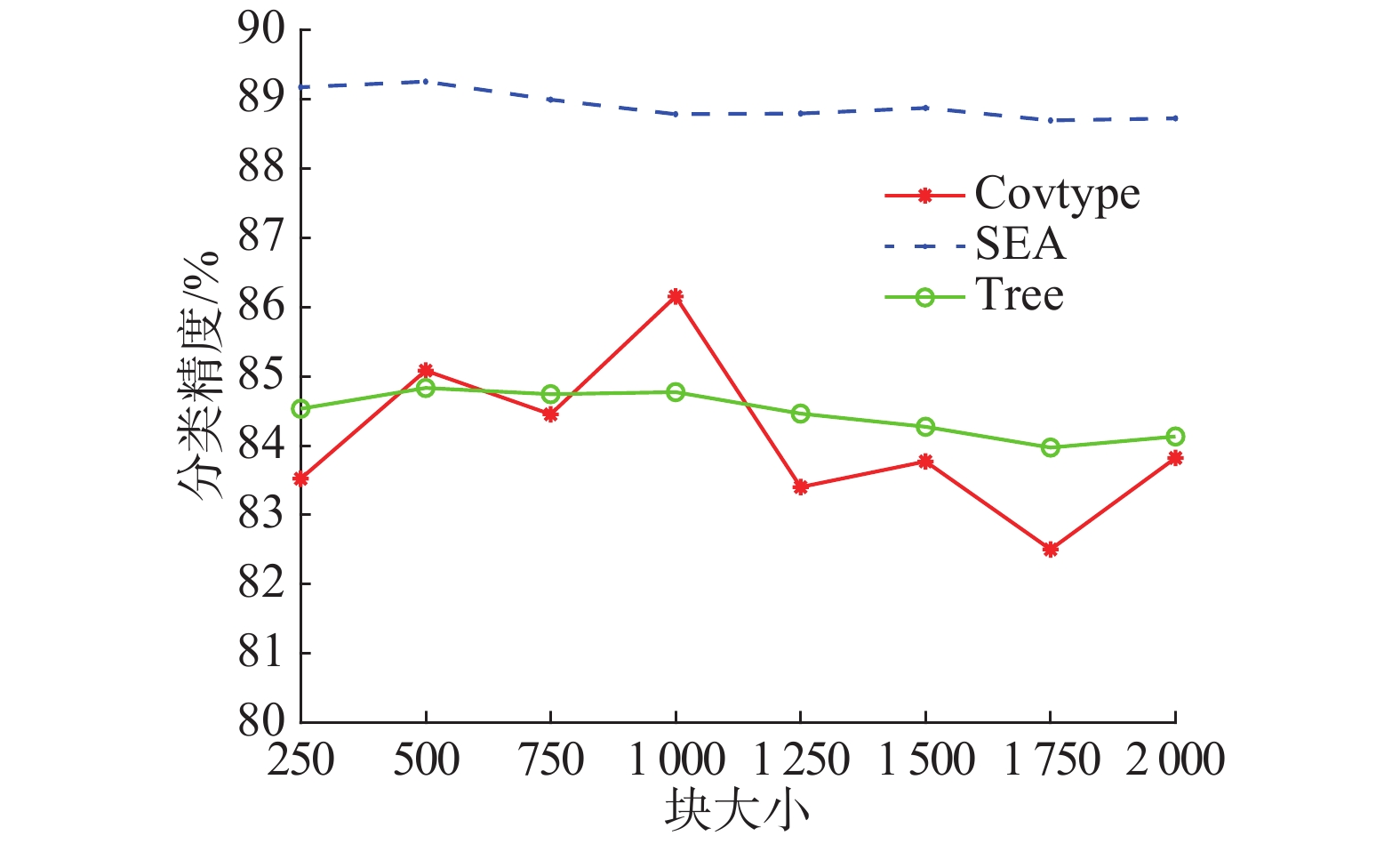Download: 图 1 不同数据块大小对算法的影响 Fig. 1 Effect of data chunk size

3.2.2 不同方法对比表 1 算法平均精度对比表 Tab.1 Contrast of different algorithms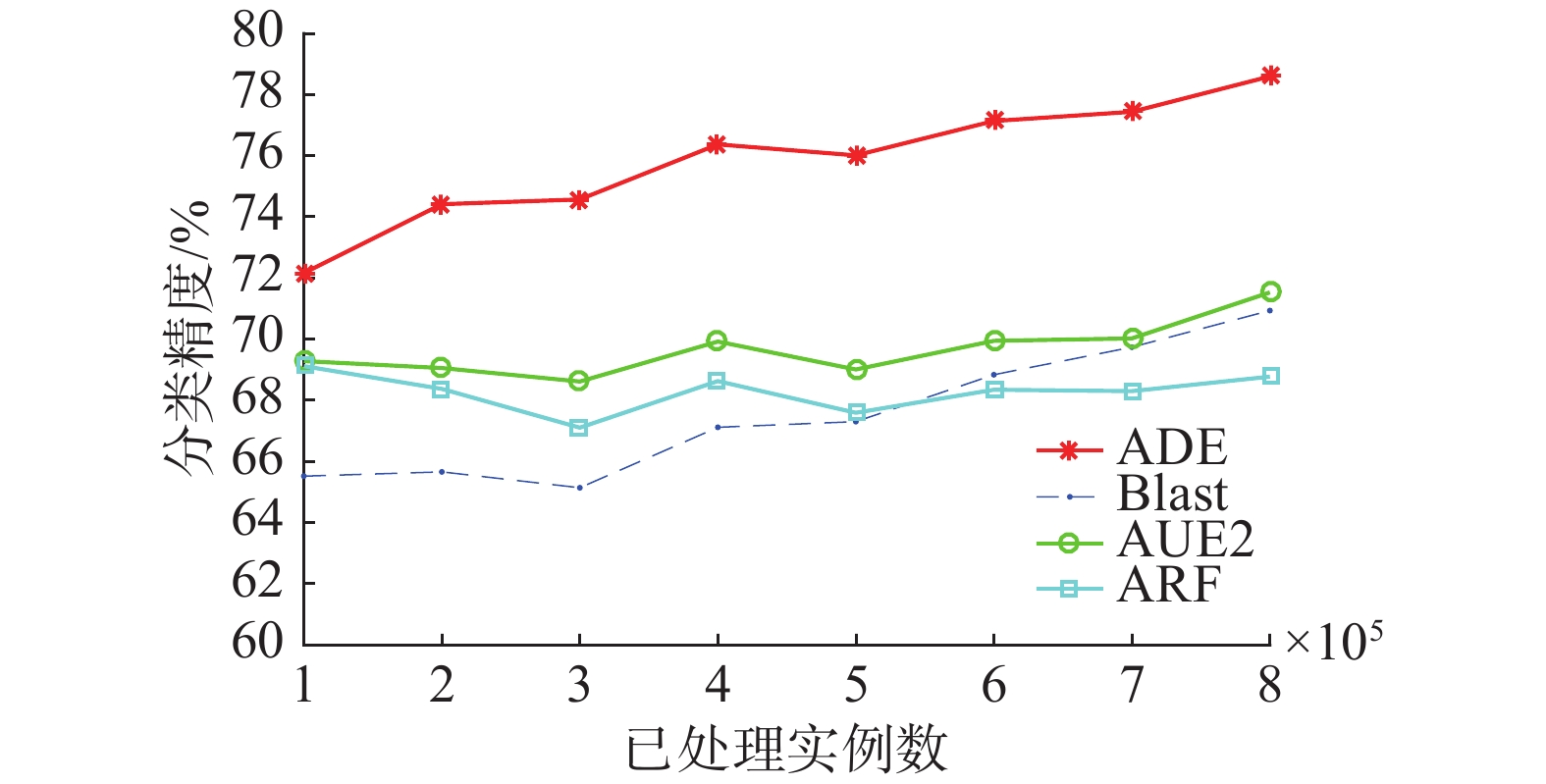Download: 图 2 4种算法在Poker数据集上分类精度对比 Fig. 2 Accuracy contrast of 4 algorithms on Poker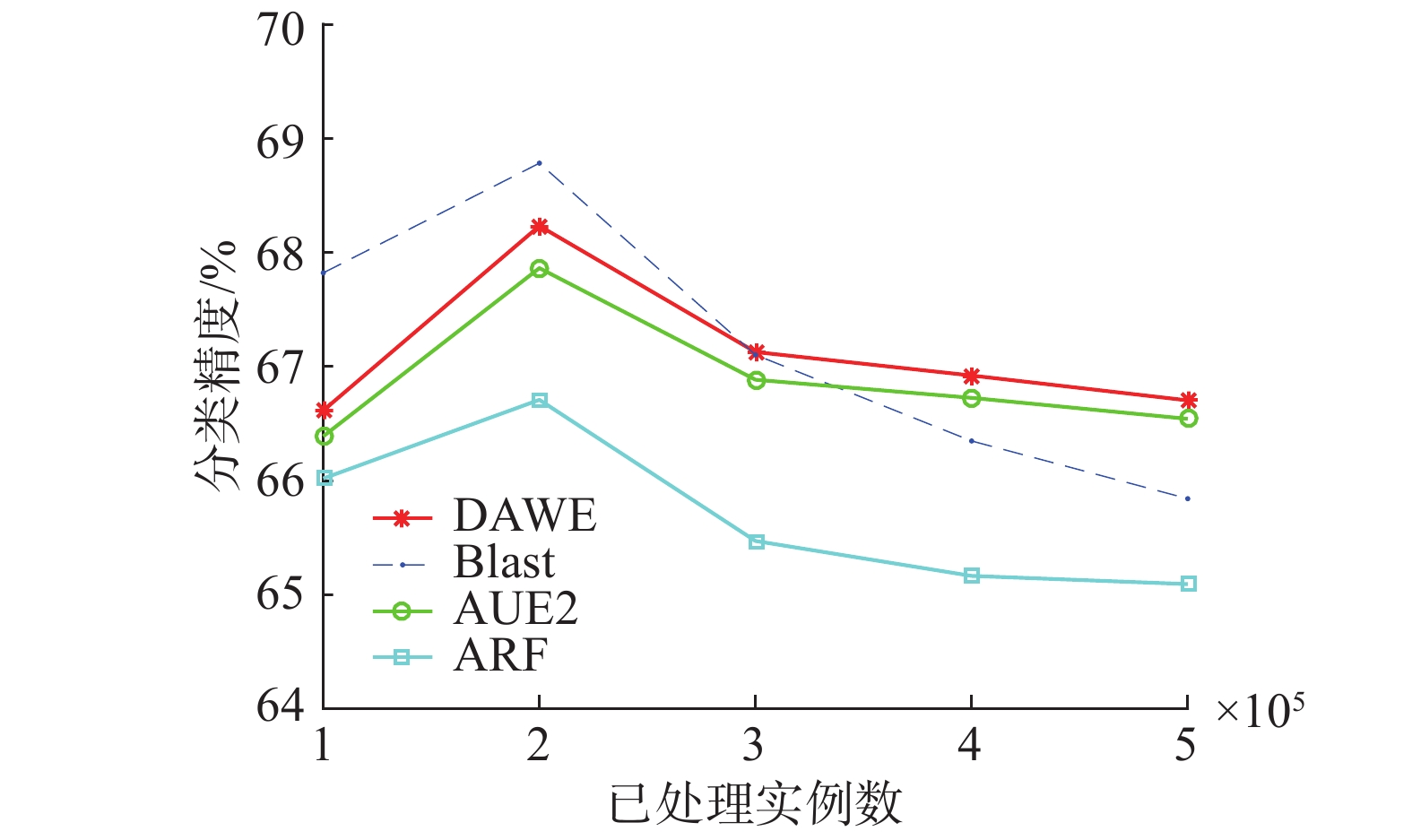Download: 图 3 4种算法在Airlines数据集上分类精度对比 Fig. 3 Accuracy contrast of 4 algorithms on Airlines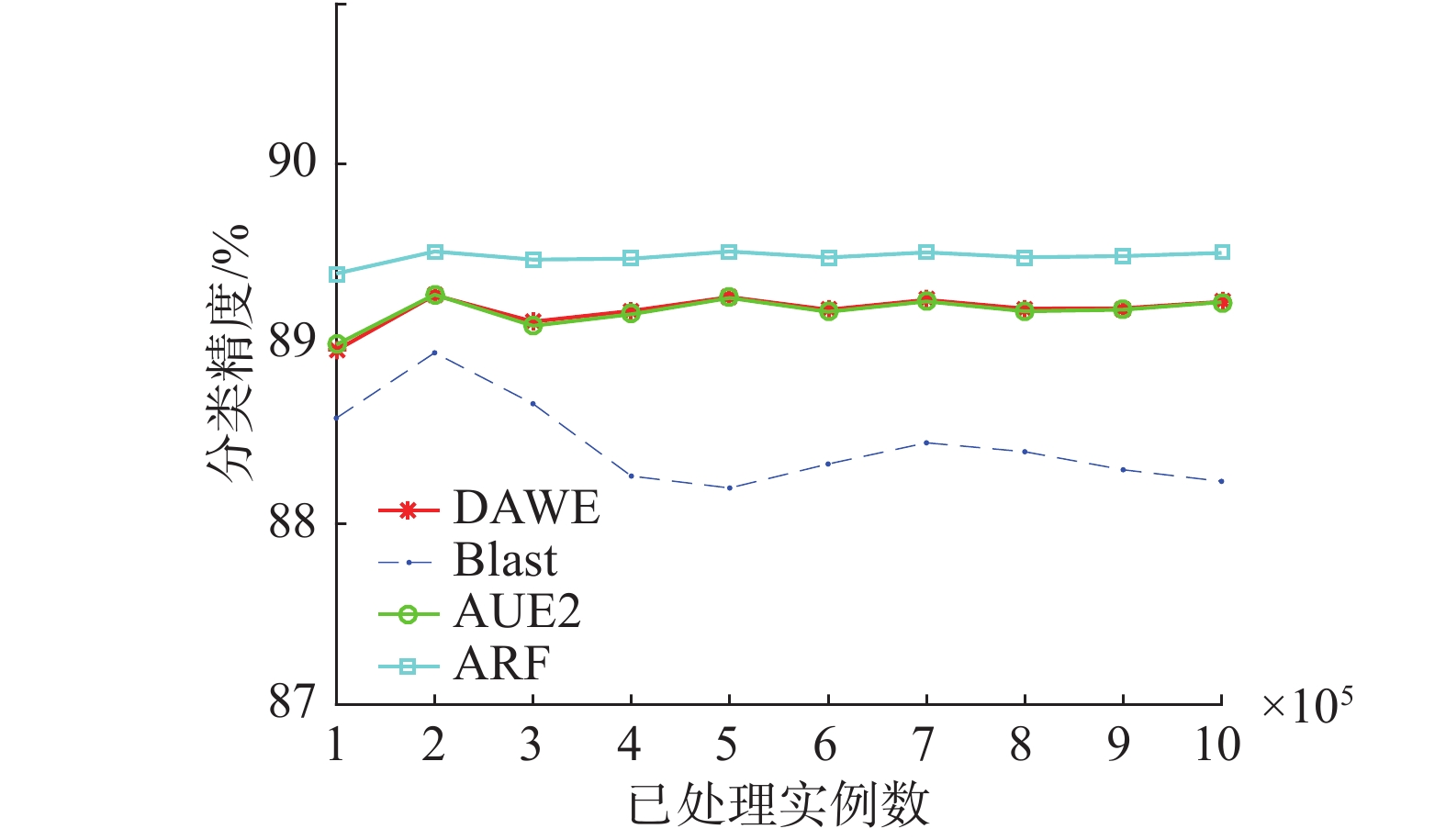Download: 图 4 4种算法在SEA数据集上分类精度对比 Fig. 4 Accuracy contrast of 4 algorithms on SEA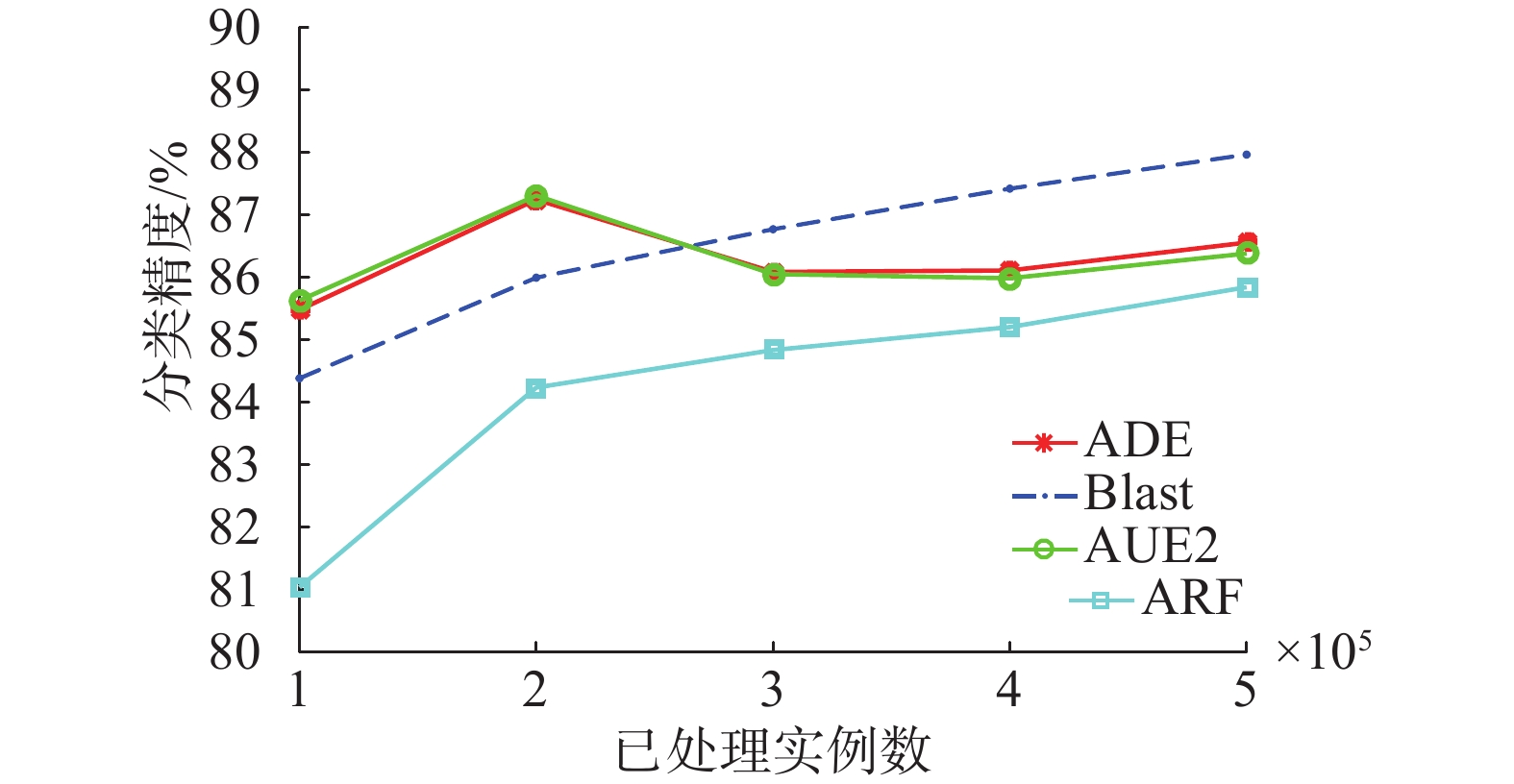Download: 图 5 4种算法在Covertype数据集上分类准确度对比 Fig. 5 Accuracy contrast of 4 algorithms on Covertype
3.2.3 α值设置对算法性能影响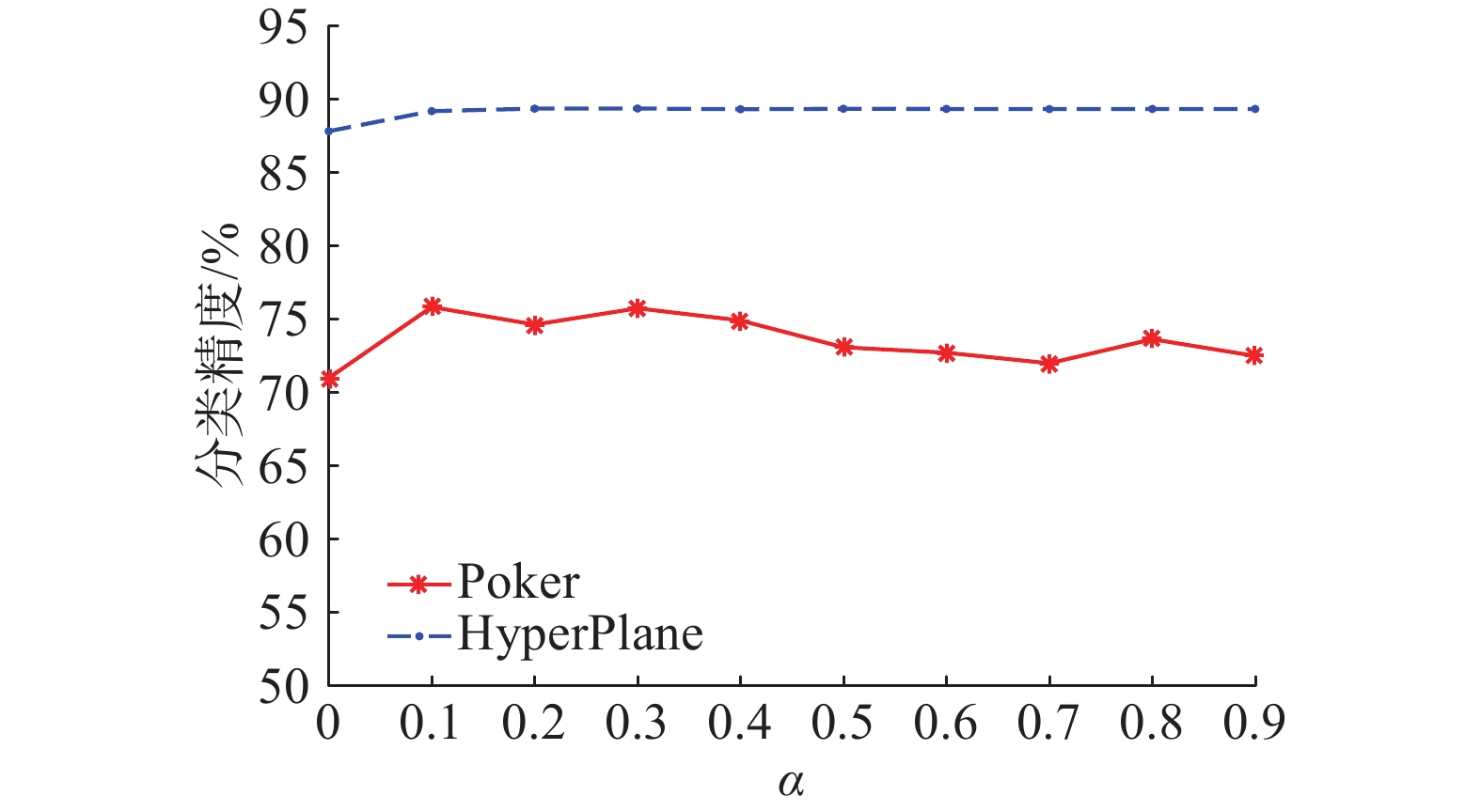Download: 图 6 不同α值对平均分类精度的影响 Fig. 6 Effect of different α on average accuracy

4 结束语

  GOMES H M, BARDDAL J P, ENEMBRECK F, et al. A survey on ensemble learning for data stream classification[J]. ACM computing surveys, 2017, 50(2): 23. (0)  BRZEZINSKI D, STEFANOWSKI J. Reacting to different types of concept drift: the Accuracy Updated Ensemble algorithm[J]. IEEE transactions on neural networks and learning systems, 2014, 25(1): 81-94. DOI:10.1109/TNNLS.2013.2251352 (0)  PIETRUCZUK L, RUTKOWSKI L, JAWORSKI M, et al. How to adjust an ensemble size in stream data mining[J]. Information sciences, 2017, 381: 46-54. DOI:10.1016/j.ins.2016.10.028 (0)  孙宇. 针对含有概念漂移问题的增量学习算法研究[D]. 合肥: 中国科学技术大学, 2017. SUN Yu. Incremental learning algorithms with concept drift adaptation[D]. Hefei: University of Science and Technology of China, 2017. http://cdmd.cnki.com.cn/Article/CDMD-10358-1017071234.htm (0)  SUN Yu, TANG Ke, ZHU Zexuan, et al. Concept drift adaptation by exploiting historical knowledge[J]. IEEE transactions on neural networks and learning systems, 2018, 29(10): 4822-4832. DOI:10.1109/TNNLS.2017.2775225 (0)  VAN RIJN J N, HOLMES G, PFAHRINGER B, et al. Having a Blast: meta-learning and heterogeneous ensembles for data streams[C]//Proceedings of the 2015 IEEE International Conference on Data Mining. Atlantic City, USA, 2015: 1003–1008. (0)  CHANDRA A, CHEN Huanhuan, YAO Xin. Trade-off between diversity and accuracy in ensemble generation[M]//JIN Yaochu. Multi-Objective Machine Learning. Berlin Heidelberg: Springer, 2006: 429–464. (0)  LI Ye, XU Li, WANG Yagang, et al. A new diversity measure for classifier fusion[M]//WANG F L, LEI Jingsheng, LAU R W H, et al. Multimedia and Signal Processing. Berlin Heidelberg: Springer, 2012: 396–403. (0)  孙博, 王建东, 陈海燕, 等. 集成学习中的多样性度量[J]. 控制与决策, 2014, 29(3): 385-395. SUN Bo, WANG Jiandong, CHEN Haiyan, et al. Diversity measures in ensemble learning[J]. Control and decision, 2014, 29(3): 385-395. (0)  BIFET A, HOLMES G, KIRKBY R, et al. MOA: massive online analysis[J]. Journal of machine learning research, 2010, 11(5): 1601-1604. (0)  STREET W N, KIM Y S. A streaming ensemble algorithm (SEA) for large-scale classification[C]//Proceedings of the 7th ACM SIGKDD International Conference on Knowledge Discovery and Data Mining. San Francisco, USA, 2001: 377–382. (0)  GOMES H M, BIFET A, READ J, et al. Adaptive random forests for evolving data stream classification[J]. Machine learning, 2017, 106(9/10): 1469-1495. (0)